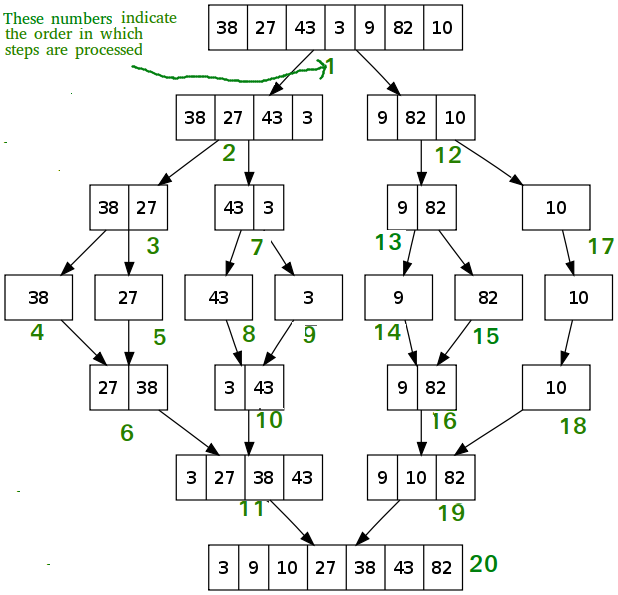# Divide and Conquer

• Last Updated : 01 Dec, 2018

Divide and Conquer is an algorithmic paradigm. A typical Divide and Conquer algorithm solves a problem using following three steps.

1. Divide: Break the given problem into subproblems of same type.
2. Conquer: Recursively solve these subproblems
3. Combine: Appropriately combine the answers

A classic example of Divide and Conquer is Merge Sort demonstrated below. In Merge Sort, we divide array into two halves, sort the two halves recursively, and then merge the sorted halves.### Recent Articles on Divide and Conquer

Topics :

Standard Algorithms :

Binary Search Based :

Misc :